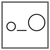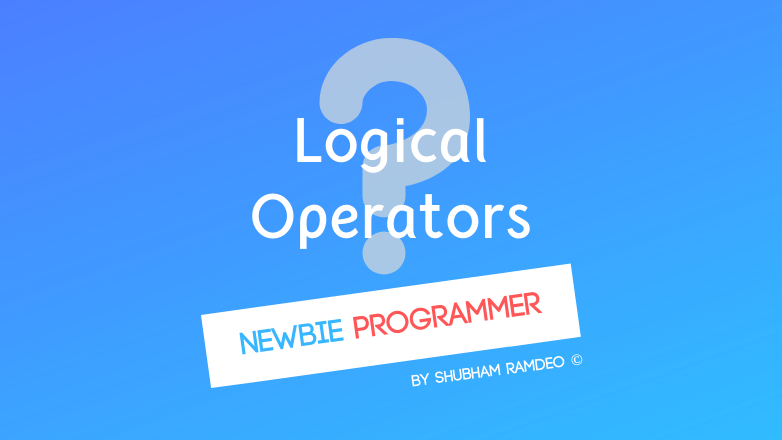Fuddlepixel coz tech is fun();

# Logical Operators

Let us discuss the logical operators through which we can perform logical operations in the C programming language, that makes coding fun !

By Shubham Ramdeo
28 August 2015 · 5 mins read
# `c`  `newbie`How can a computer performs mathematics ? It is the arithmetic operators !

Welcome back to the Newbie Programmer Series. In the last post we have learned what are relational operators and how to use them wisely. The most important thing that they gives zeros and ones as answers and not do any numerical calculations. If they find the relation true, the 1 otherwise 0.

In this part, we will learn what are the logical operators and their use within coding. If you are new to this series, please go to the index ( click here ) and check out all the previous parts so that you can easily understand what I am talking about here.

Also for this one, I have written a special article : Inside Logic Gates, The electronic logic.  Before going further, you must go to that link to learn how logic actually works with electronic devices.

## The Need of logic. Connecting Relations

I tried to write the definition of logical operators but got something like this “The Logical operators are the operators which defines the logic between two operands” !?!?! Anyways, This series is for simplicity. Therefore we will understand the logical operations through their need. A very important need more than relative operators.

The need of logical operation is the cause for birth of modern electronics. I had discussed it separately. This is programming series not electronics. But it’s needed so you can go here to learn.

Suppose we are using a computer to filter and send Birthday party Invitation to all your contacts. But the Invitation will be sent only to the people who are:

1. Above 13 years of age
2. Less than 35 years of age
3. Unmarried
4. Not your sisters
5. Either of Science Teacher or Maths Teacher ( not both )

So in a HYPOTHETICAL computer, you can give it a command:

``````SEND EMAIL TO:
(age > 13) AND (age < 35)
NOT { (married) AND (sisters) }
(science teacher) OR (maths teacher)
``````

The computer will list out the people which met the above criteria and will send the mail.

But to make such a thing we had to use some operators AND OR NOT. These three are called as logical operators. They provide the sense of choices to the program.

Now I think the above example is enough for an intro. Lets take a look to the programming stuff.

## Adding logic to code

Unlike the arithmetic operators, Logical operators DO NOT give any answers. They just check and give 1 for TRUE and 0 for FALSE.

There working is like :

(relational operation) LOGICAL OPERATOR (relational operation).

At basic level, we have three logical operators in C :

#### AND “ && “

To proceed when both the conditions are TRUE. If both the operands are non-zero, then condition becomes true.

1 && 1 TRUE, all other FALSE

( 2 > 1 ) && ( 6 < 10 )  is TRUE as both condition is true ( 2 > 1 ) && ( 6 < 1 )  is FALSE as one of the condition is false ( 1 > 2 ) && ( 6 < 1 ) is FALSE as both conditions are false. Both the conditions need to be true with the and operator.

#### OR “ || “

To proceed when at least one of the conditions is TRUE. If any of the two operands is non-zero, then condition becomes true.

 **0 0 FALSE, all other TRUE **

( 2 > 1 ) && ( 6 < 10 )  is TRUE as both condition is true ( 2 > 1 ) && ( 6 < 1 )  is TRUE as one of the condition is true ( 1 > 2 ) && ( 6 < 1 ) is FALSE as both conditions are false.

#### NOT “ ! “

To proceed when the condition is false. If the condition is false, then it gives true.  It reverses the logic. If the condition is true, it make it false. Sometimes it makes some confusions. So I would say, take it as a LIE DETECTOR. Blinks only when a lie id detected.

!(1) FALSE , !(0) TRUE

! ( 2 > 1 ) is false ( no lie detected. )

! ( 10 < 3 ) is true ( lie )

! ( ( 2 > 1 ) && ( 6 < 10 ) ) is false as the inner condition is true and NOT operator reversed it. Or, you can say NO LIE DETECTED.

 And so these were the basic logical operators of C. Why basic because you can connect them to make beautiful/complex logic systems. eg. “A && ( B ! ( B A ) )” just an example. I myself don’t know the meaning !

### Example:

Now we need to do an example to see all these practically. I have designed a great program that will help you check each of the above operators very easily. Just as we did with the relational operators.

``````#include <stdio.h>
main()
{
printf("logic checker \n");

//change the value of variables
int A = 1;
int B = 2;
while (( A < B ) && ( A < 10)) //change everything
{
printf("It is correct ! \n");
return(0); //to exit
}
printf("Not correct \n");
return(0); //to exit
}
``````

How to use it ? Simple. Change the value of the variables as you want. Change the operator in the while loop to that one you want to check. If the logic between the relations you have set is TRUE, the while loop will work and you will get printed “It is correct !” and then the program will exit. If the logic is NOT TRUE, the while loop will be skipped. You will get printed “Not Correct” and the program will exit. That’s it for today. You can make your own programs and then send me. I will post your programs for you. Play more with the above operators. I will meet you in the next post with other Operators ! Stay Connected.

Please share this post as much as you can so that we can get connected to more geeks who wants to learn programming but have no clue. Thanks for reading :) (c)Shubham Ramdeo, All rights reserved.

##### Update me weekly ✉

Subscribe below and we’ll send you a weekly email summary of all new Code tutorials. Never miss out on learning about the next big thing.

#### Shubham RamdeoShubham Ramdeo is the founder of Fuddlepixel. He is a geek, a writer, involved with many technical and humanity projects.

Meet me!

#### Recent#### Related

`c`

`newbie`

Top